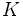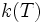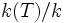# Steinitz theorem

Suppose$k$ is a field and$K$ is a field containing$k$. Then, there exists a subset$T$ of$K$, such that:
•$T$ is an algebraically independent subset of$K$; in other words the field of fractions$k(T)$ embeds inside$K$
•$K$ is algebraic over$k(T)$
Since the extension$k(T)/k$ is purely transcendental and the extension$K/k(T)$ is algebraic, Steinitz theorem can be reformulated as: every field extension can be expressed as an algebraic extension of a purely transcendental extension